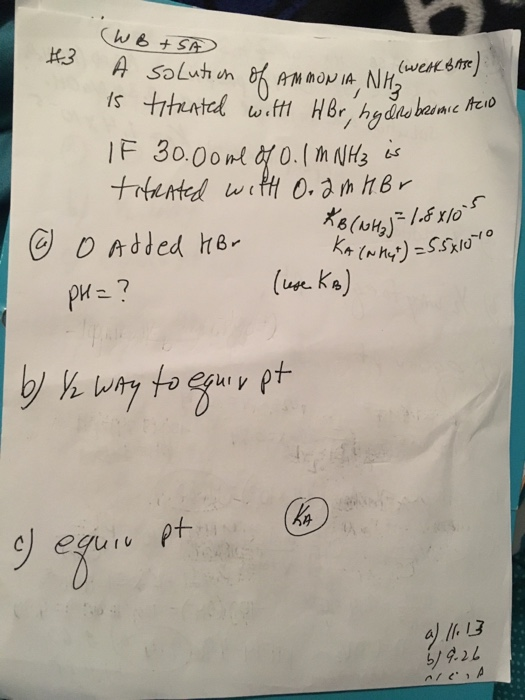# Question & Answer: I'm having trouble figuring out titration for weak base/strong acid. The answers are in the…..I’m having trouble figuring out titration for weak base/strong acid. The answers are in the corner I just have to show how to get them. Please help!

Added he 10 (une. Ka) 2 Ki

a) The reaction of NH3 with water is as below:

NH3 (aq) + H2O (l) ——-> NH4+ (aq) + OH (aq)

Don't use plagiarized sources. Get Your Custom Essay on
Question & Answer: I'm having trouble figuring out titration for weak base/strong acid. The answers are in the…..
GET AN ESSAY WRITTEN FOR YOU FROM AS LOW AS \$13/PAGE

Kb = [NH4+][OH]/[NH3]

===> 1.8*10-5 = (x).(x)/(0.1 – x)

Use small x approximation. Since Kb is small, we can assume x << 0.1 M and hence,

1.8*10-5 = x2/0.1

===> x2 = 1.8*10-6

===> x = 1.3416*10-3

[OH] = 1.3416*10-3 M and hence pOH = -log [OH] = -log (1.3416*10-3) = 2.8724

Therefore, pH = 14.00 – pOH = 14.00 – 2.8724 = 11.1276 ≈ 11.13 (ans).

b) Write down the reaction of NH3 with HBr as below:

NH3 (aq) + HBr (aq) ——-> NH4+ (aq) + Br (aq)

As per the balanced stoichiometric equation,

1 mole NH3 = 1 mole HBr = 1 mole NH4+

Half way to the equivalence point, NH3 is half neutralized and the number of moles of NH3 retained at equilibrium is equal to the number of moles of NH4+ formed.

Since the volume of the solution remains constant; [NH4+]/[NH3] = 1.

Use the Henderson-Hasslebach equation.

pOH = pKb + log [NH4+]/[NH3] = -log (Kb) + log [NH4+]/[NH3] = -log (1.8*10-5) + log (1) = 4.7447 + (0) = 4.75 (ans).

pH = 14.00 – pOH = 14.00 – 4.74 = 9.26 (ans).

c) At the equivalence point, NH3 is completely neutralized and we have NH4+ which is the conjugate acid of the weak base, NH3.

Millimoles of NH4+ formed = millimoles of NH3 present initially = (30.00 mL)*(0.1 M) = 3 mmol.

Volume of HBr required for the titration = (millimoles of NH3 present initially)/(concentration of HBr) = (3 mmol)/(0.2 M) = 15.00 mL.

Total volume of solution = (30.00 + 15.00) mL = 45.00 mL.

[NH4+] = (millimoles of NH4+)/(volume of solution) = (3 mmol)/(45.00 mL) = 0.06667 M.

The acid dissociation reaction is given as

NH4+ (aq) <=====> NH3 (aq) + H+ (aq)

Since H+ is formed, we shall use Ka.

Ka = [NH3][H+]/[NH4+] = (x).(x)/(0.06667 – x)

====> 5.5*10-10 = x2/(0.06667 – x)

Use small x approximation. Since Kb is small, we can assume x << 0.06667 M and hence,

5.5*10-10 = x2/0.06667

===> x2 = 3.66685*10-11

===> x = 6.05545*10-6

[H+] = 6.05545*10-6 M and pH = -log [H+] = -log (6.05545*10-6) = 5.2178 ≈ 5.22 (ans).